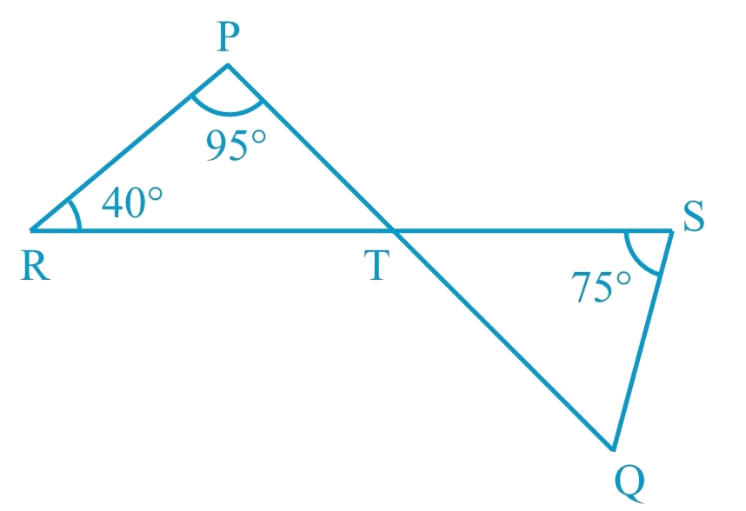# Ex.6.3 Q4 Lines and Angles Solution - NCERT Maths Class 9

## Question

In fig. below, if lines $$PQ$$ and $$RS$$ intersect at point $$T$$, such that $$\angle PRT = 40^\circ$$ ,$$\angle RPT = 95^\circ$$ and $$\angle TSQ = 75^\circ$$ , find $$\angle SQT$$ .## Text Solution

What is known?

$$\angle PRT = 40^\circ$$, $$\angle RPT = 95^\circ$$ and $$\angle TSQ = 75^\circ$$

What is unknown?

$$\angle SQT$$

Reasoning:

As we know when two line intersect each other at a point then there are two pairs of vertically opposite angles formed are equal.

Angle sum property of a triangle:

Sum of the interior angles of a triangle is $$360^\circ$$.

Steps:

Given,

$$\angle PRT = 40^\circ$$, $$\angle RPT = 95^\circ$$ and $$\angle TSQ = 75^\circ$$

In $$\Delta PRT$$

\begin{align}\angle PTR + \angle PRT + \angle RPT &= 180^\circ {\rm{ }}\\( \text{Angle sum property of a}&\text { triangle}\rm{.})\\\\\angle PTR + 40^\circ + 95^\circ &= 180^\circ \\\angle PTR &= 180^\circ - 135^\circ\\\angle{PTR} &= 45^\circ {\rm{ }}\end{align}

Now,

\begin{align}\angle QTS& = \angle PTR\quad\\( \text{Vertically }&\text {opposite angles})\\\\\angle QTS &= 45^\circ\quad \left( {\rm{i}} \right)\end{align}

In $$\Delta TSQ$$

\begin{align}\angle QTS + \angle TSQ + \angle SQT &= 180^\circ\\( \text{Angle sum property of a }&\text {triangle}.)\\\\45^\circ + 75^\circ + \angle SQT &= 180^\circ \\ [ \text{From }&( \rm{i} ) ]\\\\\angle SQT &= 180^\circ - 120^\circ \\\angle SQT &= 60^\circ \end{align}

Hence,$$\angle SQT = 60^\circ {\rm{ }}$$

Learn from the best math teachers and top your exams

• Live one on one classroom and doubt clearing
• Practice worksheets in and after class for conceptual clarity
• Personalized curriculum to keep up with school